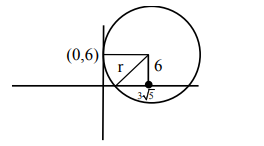# Solve this following`
Question:

Consider a circle $C$ which touches the $y$-axis at $(0,6)$ and cuts off an intercept $6 \sqrt{5}$ on the x-axis. Then the radius of the circle $\mathrm{C}$ is equal to :

1. $\sqrt{53}$

2. 9

3. 8

4. $\sqrt{82}$

Correct Option: , 2

Solution:$r=\sqrt{6^{2}+(3 \sqrt{5})^{2}}$

$=\sqrt{36+45}=9$